# Python 与列表相关的6个操作

Python

1. 将两个列表合并到一个字典中

keys_list = ['A', 'B', 'C']

values_list = ['blue', 'red', 'bold']

#  3 种方法可以将这两个列表转换为字典

# 1.使用Python zipdict函数

dict_method_1 = dict(zip(keys_list, values_list))

# 2. 使用带有字典推导式的 zip 函数

dict_method_2 = {key:value for key, value in zip(keys_list, values_list)}

# 3.循环使用zip函数

items_tuples = zip(keys_list, values_list)

dict_method_3 = {}

for key, value in items_tuples:

if key in dict_method_3:

pass

else:

dict_method_3[key] = value

print(dict_method_1)

print(dict_method_2)

print(dict_method_3)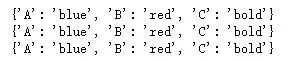2.将两个或多个列表合并为一个列表

def merge(*args, missing_val = None):

max_length = max([len(lst) for lst in args])

outList = []

for i in range(max_length):

outList.append([args[k][i] if i < len(args[k]) else missing_val for k in range(len(args))])

return outList

merge([1,2,3],['a','b','c'],['h','e','y'],[4,5,6])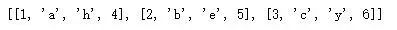3. 对字典列表进行排序

dicts_lists = [

{

Name: James,

Age: 20,

},

{

Name: May,

Age: 14,

},

{

Name: Katy,

Age: 23,

}

]

# 方法一

dicts_lists.sort(key=lambda item: item.get(Age))

# 方法二

from operator import itemgetter

f = itemgetter('Name')

dicts_lists.sort(key=f)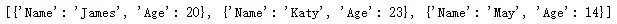4. 对字符串列表进行排序

my_list = [blue, red, green]

# 方法一

my_list.sort()

my_list = sorted(my_list, key=len)

# 方法二

import locale

from functools import cmp_to_key

my_list = sorted(my_list, key=cmp_to_key(locale.strcoll))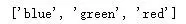5. 根据另一个列表对列表进行排序

a = ['blue', 'green', 'orange', 'purple', 'yellow']

b = [3, 2, 5, 4, 1]

sortedList =  [val for (_, val) in sorted(zip(b, a), key=lambda x: x)]

print(sortedList)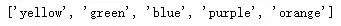6. 将列表映射到字典

mylist = ['blue', 'orange', 'green']

#Map the list into a dict using the map, zip and dict functions

mapped_dict = dict(zip(itr, map(fn, itr)))

————————————————